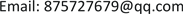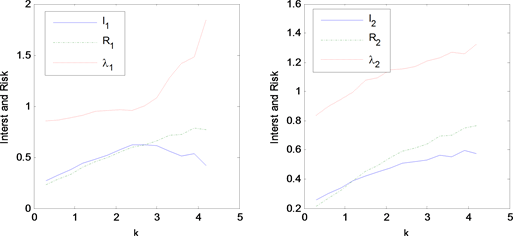﻿ 基于波动率状态转移的股票配资模型 Stock Loan Model under Volatility Regime Switching

Advances in Applied Mathematics
Vol.07 No.05(2018), Article ID:24884,6 pages
10.12677/AAM.2018.75059

Stock Loan Model under Volatility Regime Switching

Xuan Zhu, Miaomaio Xie, Yue Gao, Liling Wu

School of Mathematics and Computation Science, Huaihua University, Huaihua HunanReceived: Apr. 22nd, 2018; accepted: May 8th, 2018; published: May 16th, 2018ABSTRACT

Under Volatility Regime Switching and by using option pricing theorem, this paper establishes a mathematical model for stock loan, and then uses finite difference method to solve it. Some numerical examples illustrate that the proposed model has good efficiency at the aspect of analyzing interest rate and risk rate.

Keywords:Stock Loan, Stock Option, Option Partial Differential Equation, Finite Difference MethodCopyright © 2018 by authors and Hans Publishers Inc.1. 股票配资合同

$\text{d}{S}_{t}\left({X}_{i}\right)=\left(r-\delta \right){S}_{t}\left({X}_{i}\right)\text{d}t+{\sigma }_{i}{S}_{t}\left({X}_{i}\right)\text{d}{W}_{t},\text{\hspace{0.17em}}\text{\hspace{0.17em}}i=1,2\cdots ,d$ (1)

2. 配资收益与风险模型

$A=\left[\begin{array}{cccc}-{a}_{11}& {a}_{12}& \dots & {a}_{1d}\\ {a}_{21}& -{a}_{22}& \dots & {a}_{2d}\\ ⋮& ⋮& \ddots & ⋮\\ {a}_{d1}& {a}_{d2}& \dots & -{a}_{dd}\end{array}\right],\text{\hspace{0.17em}}\text{\hspace{0.17em}}{a}_{ij}\ge 0$ , $\sum _{j=1,j\ne i}^{d}{a}_{ij}={a}_{ii},\text{\hspace{0.17em}}i=1,2,\cdots ,d$

$h\left(T,{S}_{T}\right)={\left({S}_{T}-kq{\text{e}}^{\gamma T}-q\right)}^{+}$${\left(\cdot \right)}^{+}$ 表示取正部函数，而股民在任意t时刻的贴现收益期望为

${V}_{i}\left(t,{S}_{t}\left({X}_{i}\right)\right)={E}^{t,{S}_{t}\left({X}_{i}\right)}\left[{\text{e}}^{-r\left(T-t\right)}h\left(T,{S}_{T}\right)\right].$ (2)

$\begin{array}{l}\frac{\partial }{\partial t}{V}_{i}\left(t,S\right)+\left(r-\delta \right)S\frac{\partial }{\partial S}{V}_{i}\left(t,S\right)+\frac{1}{2}{\sigma }^{2}{S}^{2}\frac{{\partial }^{2}}{\partial {S}^{2}}{V}_{i}\left(t,S\right)-\left(r+{a}_{ii}\right){V}_{i}\left(t,S\right)\\ +\sum _{j=1,j\ne i}^{d}{a}_{ij}{V}_{j}\left(t,S\right)=0,\text{ }i=1,2,\cdots ,d\end{array}$ (3)

${V}_{i}\left(T,S\right)={\left(S-kq{\text{e}}^{\gamma T}-q\right)}^{+}.$ (4)

${V}_{i}\left(t,kq{\text{e}}^{\gamma t}\right)=0,$ (5)

${V}_{i}\left(t,\mu q\right)=\left(\mu -1\right)q-kq{\text{e}}^{\gamma t}.$ (6)

PDEs (3) (4) (5)和(6)构成多状态转移欧式看涨障碍期权。如果转移概率矩阵 $A=0$ ，状态转移模型退化为文  所描述的经典布朗运动模型。

$\stackrel{˜}{h}\left(T,{S}_{T}\right)={\left(q+kq{\text{e}}^{\gamma T}-{S}_{T}\right)}^{+}$ ，而股民在任意t时刻的贴现风险期望为

${\stackrel{˜}{V}}_{i}\left(t,{S}_{t}\left({X}_{i}\right)\right)={E}^{t,{S}_{t}\left({X}_{i}\right)}\left[{\text{e}}^{-r\left(T-t\right)}\stackrel{˜}{h}\left(T,{S}_{T}\right)\right].$ (7)

$\begin{array}{l}\frac{\partial }{\partial t}{\stackrel{˜}{V}}_{i}\left(t,S\right)+\left(r-\delta \right)S\frac{\partial }{\partial S}{\stackrel{˜}{V}}_{i}\left(t,S\right)+\frac{1}{2}{\sigma }^{2}{S}^{2}\frac{{\partial }^{2}}{\partial {S}^{2}}{\stackrel{˜}{V}}_{i}\left(t,S\right)-\left(r+{a}_{ii}\right){\stackrel{˜}{V}}_{i}\left(t,S\right)\\ +\sum _{j=1,j\ne i}^{d}{a}_{ij}{\stackrel{˜}{V}}_{j}\left(t,S\right)=0,\text{ }i=1,2,\cdots ,d\end{array}$ (8)

${\stackrel{˜}{V}}_{i}\left(T,S\right)={\left(q+kq{\text{e}}^{\gamma T}-S\right)}^{+}.$ (9)

${\stackrel{˜}{V}}_{i}\left(t,kq{e}^{\gamma t}\right)=q,\text{\hspace{0.17em}}\text{\hspace{0.17em}}{\stackrel{˜}{V}}_{i}\left(t,\mu q\right)=0.$ (10)

PDEs (8) (9) (10)构成所谓的欧式看跌障碍期权。如果已知零时刻股票价格 ${S}_{0}$ ，并给定配资率k，股

${I}_{i}={V}_{i}\left(0,{S}_{0}\right)/q,\text{\hspace{0.17em}}\text{\hspace{0.17em}}{R}_{i}={\stackrel{˜}{V}}_{i}\left(0,{S}_{0}\right)/q,\text{\hspace{0.17em}}\text{\hspace{0.17em}}{\lambda }_{i}=\frac{{\stackrel{˜}{V}}_{i}\left(0,{S}_{0}\right)}{{V}_{i}\left(0,{S}_{0}\right)},$ (11)

${I}_{i}$${R}_{i}$${\lambda }_{i}$ 都是无量纲的， ${I}_{i}$ 表示单位投入的收益， ${R}_{i}$ 表示单位投入的风险， ${\lambda }_{i}$ 则表示单位收益所面临的风险大小。 ${I}_{i}$${R}_{i}$${\lambda }_{i}$ 对配资率k较为敏感，同时与借贷利率γ有关，应该是股民最为关注的数量指标。

$\Delta t=T/M,\text{\hspace{0.17em}}\text{\hspace{0.17em}}{t}_{m}=m\Delta t,\text{\hspace{0.17em}}\text{\hspace{0.17em}}m=0,1,\cdots ,M$

$\Delta {S}^{m}=\left(\mu q-kq{\text{e}}^{\gamma {t}_{m}}\right)/N,\text{\hspace{0.17em}}\text{\hspace{0.17em}}{S}_{n}^{m}=kq{\text{e}}^{\gamma {t}_{m}}+n\Delta {S}^{m},\text{\hspace{0.17em}}n=0,1,\cdots ,N.$

${V}_{m,n}^{i}$ 为期权价值 ${V}_{i}\left(t,S\right)$ 在状态 ${X}_{i}$ ，时刻 ${t}_{m}$ 和股价为 ${S}_{n}^{m}$ 时的数值解。运用中心差分的隐式格式离散PDE (3)得到

$\begin{array}{l}\frac{{\stackrel{^}{V}}_{m,n}^{i}-{V}_{m-1,n}^{i}}{\Delta t}+\left(r-\delta \right){S}_{n}^{m-1}\frac{{V}_{m-1,n+1}^{i}-{V}_{m-1,n-1}^{i}}{2\Delta {S}^{m-1}}+\frac{1}{2}{\sigma }^{2}{\left({S}_{j}^{m-1}\right)}^{2}\frac{{V}_{m-1,n+1}^{i}-2{V}_{m-1,n}^{i}+{V}_{m-1,n-1}^{i}}{{\left(\Delta {S}^{m-1}\right)}^{2}}\\ -\left(r+{a}_{ii}\right){V}^{i}{}_{m-1,n}+\sum _{j=1,j\ne i}^{d}{a}_{ij}{V}_{m-1,n}^{j}=0,\text{ }m=M,M-1,\cdots ,1;\text{\hspace{0.17em}}n=1,2,\cdots ,N-1.\end{array}$ (12)

$\begin{array}{l}{p}_{i,m-1,n}{V}_{m-1,n-1}^{i}+{q}_{i,m-1,n}{V}_{m-1,n}^{i}+{c}_{i,m-1,n+1}{V}_{m-1,n}^{i}\\ +\sum _{j=1,j\ne i}^{d}{l}_{i,j}{V}_{m-1,n}^{j}={\stackrel{^}{V}}_{m,n}^{i},\text{\hspace{0.17em}}\text{\hspace{0.17em}}m=1,2,\cdots ,N-1,\end{array}$ (13)

$\begin{array}{l}{p}_{i,m-1,n}=\left(r-\delta \right){S}_{n}^{m-1}\Delta t-\frac{{\sigma }_{i}^{2}{\left({S}_{n}^{m-1}\right)}^{2}\Delta t}{2{\left(\Delta {S}^{m-1}\right)}^{2}},\\ {q}_{i,m-1,n}=1+\frac{{\sigma }_{i}^{2}{\left({S}_{n}^{m-1}\right)}^{2}\Delta t}{{\left(\Delta {S}^{m-1}\right)}^{2}}+\left(r+{a}_{ii}\right)\Delta t,\\ {c}_{i,m-1,n}=-\left(r-\delta \right){S}_{n}^{m-1}\Delta t-\frac{{\sigma }_{i}^{2}{\left({S}_{n}^{m-1}\right)}^{2}\Delta t}{2{\left(\Delta {S}^{m-1}\right)}^{2}},\text{\hspace{0.17em}}{l}_{i,j}={a}_{ij}\Delta t.\end{array}$

${V}_{M,n}^{i}={\left({S}_{n}^{M}-kq{\text{e}}^{\gamma T}-q\right)}^{+},\text{ }{V}_{m-1,0}=0,\text{ }{V}_{m,N}=\left(\mu -1\right)q-kq{\text{e}}^{\gamma {t}_{m}},$

$\begin{array}{l}{B}_{i}=Diag\left({p}_{i,m-2,n},{q}_{i,m-2,n},{c}_{i,m-2,n}\right),\\ {f}_{i}={\left[{\stackrel{^}{V}}_{m,1}^{i},{\stackrel{^}{V}}_{m,2}^{i},\cdots ,{\stackrel{^}{V}}_{m,N}^{i}-\left(\mu -1\right)q-kq{\text{e}}^{\gamma {t}_{m}}\right]}^{\text{T}}\end{array}$

3. 数值模拟与分析Table 1. Impact of interest and risk for different allocation rateFigure 1. Impact of interest and risk for different allocation rate. Left sub-figure for regime-1 and right sub-figure for regime-2

2016年度湖南省大学生研究性学习和创新性实验计划项目。

Stock Loan Model under Volatility Regime Switching[J]. 应用数学进展, 2018, 07(05): 495-500. https://doi.org/10.12677/AAM.2018.75059

1. 1. Lu, X.P. and Putri, E.R.M. (2015) Semi-Analytic Valuation of Stock Loans with Finite Maturity. Communications in Nonlinear Science and Numerical Simulation, 27, 206-215.
https://doi.org/10.1016/j.cnsns.2015.03.007

2. 2. Lu, X.P. and Putri, E.R.M. (2016) Finite Maturity Margin Call Stock Loans. Operations Research Letters, 44, 12-18.
https://doi.org/10.1016/j.orl.2015.10.007

3. 3. Zhou, Z. and Gao, X. (2016) Numerical Methods for Pricing American Options with Time Fractional PDE Models. Mathematical Problems in Engineering, 2016, Article ID: 5614950, 8 p.

4. 4. Zhou, Z. and Ma, J. (2016) Lattice Boltzmann Methods for Solving PDEs of Exotic Option Pricing. Frontiers of Mathematics in China, 11, 237-254.
https://doi.org/10.1007/s11464-015-0500-0

5. 5. 吴红英, 朱萱, 杨令. 股票配资的收益与风险控制模型[J]. 怀化学院学报, 2017(11): 37-40.

6. 6. Shreve, S.E. (2003) Stochastic Calculus for Finance I: The Binomial Asset Pricing Model. Springer, New York.

7. 7. Shreve, S.E. (2004) Stochastic Calculus for Finance II: Continuous-Time Models. Springer Press, New York.
https://doi.org/10.1007/978-1-4757-4296-1